Navigation is one of the excellent creations by using SWF Quicker; it provides enhancements that make it easier for you to write more robust scripts using the ActionScript language. In this tutorial, I focus on telling you how to make a flexible navigation menu in details.

Series: SWF Quicker 2.0

Zooming is inhibited in the above SWF. You can make it greater in size to get the full dragging effect while you place it in Web page.

Since the designing task that making six buttons and placing them properly are easy for you, I will focus on explaining the codes but not the designing process. For more information, please refer to source file at the end of this tutorial I provide for you.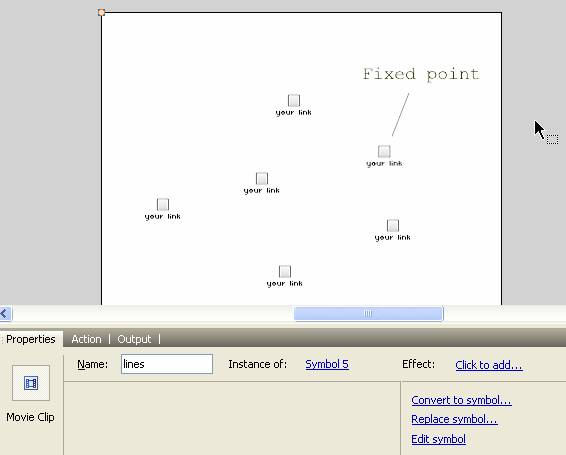I want to share my idea formed before I get down to making this navigation menu with all of you. Let us back to observe this chain motion. The other buttons keep a distance and an angle with the one you press and drag. How to make it? First, enter a0, a1, a2… as instance name of the movie clip to contact corresponding number 0, 1, 2, 3… and then we can control the contact between the buttons around by plusing or minusing the numbers. That is we called circulation. Many skills are included in the following codes I show you.

 Code: stop(); fscommand("allowscale", false); //the following customize the event when you press and release the button. this.dragging is to indicate that the button is pressed down; //it is to avoid the collision between mouse control and computer control; //_root.dr is to indicate one of the buttons is pressed down in main scene; function doDrag() {   this.startDrag();   this.dragging = true;   _root.dr = true;   this.pres = true;   this.gotoAndStop(2); } function noDrag() {   stopDrag();   this.dragging = false;   _root.dr = false;   this.pres = false;   this.gotoAndStop(1); } //change icon when cursor over; function showme() {   this.gotoAndStop(2); } //back a random coordinate. Use the distance between it and original coordinate which is less than 15 to make a slight shake; function rando(x, y) {   six = Math.random()*30+1+(x-15);   siy = Math.random()*30+1+(y-15); } //following is to control move; function makemove() { //it can be divided into several circs: one is that no button is pressed down in main movie. Write an original position; //generate random move by random function and back to original position; if (!_root.dr) {   if (random(80) == 1) {//It move it self even if you don’t touch it;    var s = random(6);    rando((_root["a"+s].oldx), _root["a"+s].oldy);    _root["a"+s]._x = six;    _root["a"+s]._y = siy;   } //the following is flexible move formula. First to talk about “k”, which is to partition the distance between current position and target point. “vx” increases gradually when “dx” decreases. “dx” changes to negative when “vx” exceeds target point.  Then”vx” decreases gradually and back to move to make a ceaseless shake;   var dx = this.oldx-this._x;   var dy = this.oldy-this._y;   this.vx += dx*k;   this.vy += dy*k;   this._x = this._x+this.vx;   this._y = this._y+this.vy;   this.vx = this.vx*damp;   this.vy = this.vy*damp; //one circ is that one button is pressed down but not the current button; } else if (!this.dragging && _root.dr) {   mytxt = new String(this._name);   namenumber = Number(mytxt.charAt(1)); //it is not button 0 or button 5;   if (namenumber != 0 && namenumber != 5) {    var xdis = this._x-_root["a"+(namenumber+1)]._x;    var ydis = this._y-_root["a"+(namenumber+1)]._y;    var ang = Math.atan2(ydis, xdis); // design a target which distance itself 90 and approach to the near buttons including the one before and after it;    var tarx = _root["a"+(namenumber+1)]._x+Math.cos(ang)*90;    var tary = _root["a"+(namenumber+1)]._y+Math.sin(ang)*90;    this.vx += (tarx-this._x)*k;    this.vy += (tary-this._y)*k;    this.vx = this.vx*damp;    this.vy = this.vy*damp;    var xdis = this._x-_root["a"+(namenumber-1)]._x;    var ydis = this._y-_root["a"+(namenumber-1)]._y;    var ang = Math.atan2(ydis, xdis);    var tarx = _root["a"+(namenumber-1)]._x+Math.cos(ang)*90;    var tary = _root["a"+(namenumber-1)]._y+Math.sin(ang)*90;    this.vx += (tarx-this._x)*k;    this.vy += (tary-this._y)*k;    this.vx = this.vx*damp;    this.vy = this.vy*damp;    this._x += this.vx;    this._y += this.vy; //the circs of button 5. We take button 0 as a fixed point which is always back to original position;   } else if (namenumber == 5) {    var xdis = this._x-_root.a4._x;    var ydis = this._y-_root.a4._y;    var ang = Math.atan2(ydis, xdis);    var tarx = _root.a4._x+Math.cos(ang)*90;    var tary = _root.a4._y+Math.sin(ang)*90;    this.vx += (tarx-this._x)*k;    this.vy += (tary-this._y)*k;    this.vx = this.vx*damp;    this.vy = this.vy*damp;    var xdis = this._x-_root.a0._x;    var ydis = this._y-_root.a0._y;    var ang = Math.atan2(ydis, xdis);    var tarx = _root.a0._x+Math.cos(ang)*90;    var tary = _root.a0._y+Math.sin(ang)*90;    this.vx += (tarx-this._x)*k;    this.vy += (tary-this._y)*k;    this.vx = this.vx*damp;    this.vy = this.vy*damp;    this._x += this.vx;    this._y += this.vy;   }  }  }  //initial number here; k = 0.4; damp = 0.7; numFeet = 5; forbid = 0; // bind six transparent buttons to respond dragging, the coordinates is corresponding to six buttons one by one.; for (i=0; i<=5; i++) {   _root.lines.attachMovie("b", "a"+i, -i);   _root.lines["a"+i]._x = _root["a"+i]._x;   _root.lines["a"+i]._y = _root["a"+i]._y;   _root["a"+i].oldx = _root["a"+i]._x;   _root["a"+i].oldy = _root["a"+i]._y;   _root["a"+i].pres = false; } //add event for 6 button movie clip; for (i=0; i<=5; i++) {  _root["a"+i].onRollOver = showme;  _root["a"+i].onPress = doDrag;  _root["a"+i].onRelease = noDrag;  _root["a"+i].onReleaseOutside = noDrag;  _root["a"+i].onEnterFrame = makemove;  _root["a"+i].follow = 0; _root["a"+i].vx =0;  _root["a"+i].vy =0; } //follow the coordinates of 6 button movie clip and draw a line between them; _root.lines.onEnterFrame = function() {  _root.lines.clear();  _root.lines.lineStyle(1, 0, 50);  i = 0;  while (i<=numFeet) {   if (i != 5) {    _root.lines.moveTo(_root["a"+i]._x, _root["a"+i]._y);    _root.lines.lineTo(_root["a"+(i+1)]._x, _root["a"+(i+1)]._y);   } else if (i == 5) {    _root.lines.moveTo(_root.a5._x, _root.a5._y);    _root.lines.lineTo(_root.a0._x, _root.a0._y);   }   i++;  } };

Dragging effect can be made by pressing the movie clip "button"; adoptive effect can be made by rolling over a movie clip "button". We use a transparent movie clip to detect the above two effects, one more needs to kown, function “hitText” is key function here. The following codes are added to the transparent movie clip.

 Code: onClipEvent (load) {   this.oldx = _parent._x;   this.oldy = _parent._y; } onClipEvent (enterFrame) {   this.dx = (_parent._x-this.oldx)*(_parent._x-this.oldx)+(_parent._y-this.oldy)*(_parent._y-this.oldy);   if (_root.forbid == 0 || _root.forbidname == _parent._name) {    if (_parent.hitTest(_root._xmouse, _root._ymouse, true)) {       _root.forbid = 1;       _root.forbidname = _parent._name;       t = substring(_parent._name, 2, 1);       this.ok = 1;       if (this.dx>=20000 && _root["a"+t].pres == false) {          _parent._x = this.oldx;          _parent._y = this.oldy;          _root.dr = false;          _root["a"+t].dragging = false;          _root.forbidname = _parent._name;          _root.forbid = 0;          _root["a"+t].gotoAndStop(1);        } else {          _root.dr = true;          _root["a"+t].dragging = true;          _parent._x = _root._xmouse;          _parent._y = _root._ymouse;          _root["a"+t]._x = _root._xmouse;          _root["a"+t]._y = _root._ymouse;        }       } else {        if (_root["a"+t].pres == false) {           if (this.ok) {            _parent._x = this.oldx;            _parent._y = this.oldy;            _root.dr = false;            _root["a"+t].dragging = false;            this.ok = 0;            _root.forbidname = _parent._name;            _root.forbid = 0;            _root["a"+t].gotoAndStop(1);           }         }       }    } }

The function of codes above are: the buttons are following cursor when your cursor is close to button, just like you have already touched the button and to drag it. The farther the cursor is away from the original coordinates, the more weak the adsorption is.

Now, I will talk something about the rule of flexibility.

Look at the following codes first:

 Code: onClipEvent (load) {   ax=0.5   vx=2 } onClipEvent (enterFrame) {   vx += ax;   _x += vx; }

Codes above are simple, movie clip speeds up ceaselessly. The accelerated number is decided by “ax”.

I give you an example to help you more understand flexibility. Do you remember the single pendulum always mentioned in physics? The acceleration decreases gradually when the single pendulum moves from initial position to midpoint and at the same time the velocity is increased gradually. The swing will decrease gradually if single pendulum confronts resistance.

What we need to do is to change the acceleration in the above codes. Continue taking single pendulum as an analogy, the acceleration decreases when single pendulum pass the balance point.

The rule is easy to understand: the bigger the distance between single pendulum and balance point is; the larger accelerate is. We can get: ax=(tx-_x)*k.

tx is target position, or we called balance position. Because of resistance, single pendulum can finally stop at the balance position. We add a damp coefficient here:

 Code: onClipEvent (enterFrame) { //original formula: //ax = (tx-_x)*k; //vx += ax; vx += (tx-_x)*k;   vx*=damp;   _x += vx; }

Completed codes for single pendulum are as follows:

 Code: onClipEvent(load) {    tx = _x;    ty = _y;    trace(tx);    draged = false;    k = 0.3;    damp = 0.8;    vx = 0;    vy = 0; } on(press) {    this.startDrag(true);    draged = false; } on(release) {    this.stopDrag();    draged = true; } onClipEvent(enterFrame) {    if (draged) {       vx += (tx-_x)*k;       vy += (ty-_y)*k;       vx = vx*damp;       vy = vy*damp;       _x+= vx;       _y+= vy;    } }Sothink is a trademark of SourceTec Software Co., LTD.•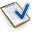Support
•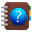Tutorials
•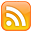Subscribe
•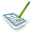Blog
•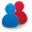Forum
•Affiliate